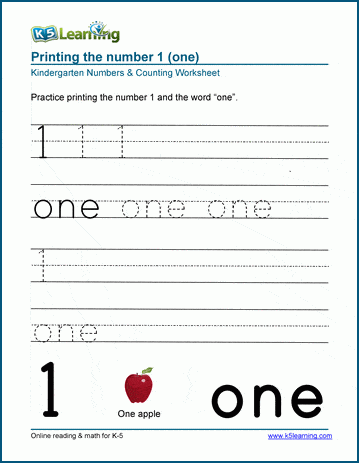# Numbers worksheets

Our learning numbers worksheets introduce kids to recognizing and printing numbers. Numbers in words and ordinal numbers are also introduced, as is the concept of odd and even numbers.

Kindergarten learning numbers worksheets

Kindergarten odd / even numbers worksheets

Kindergarten ordinal numbers worksheets

Topics include:

## Kindergarten learning numbers worksheets

• Recognizing numbers from 1-10
• Printing numbers (as numbers and words) 1-20
• Matching numbers to their words
• Coloring by number

## Kindergarten odd / even numbers worksheets

• Is the number odd or even?
• Identify even vs. odd numbers

## Kindergarten ordinal numbers (1st, 2nd ...) worksheets

• Matching ordinal numbers to their position
• Match ordinal numbers to their words
• Write ordinal numbers in words

• Read the number and circle the correct number of objects
• Count objects and write the number (1-20)
• Number charts
• Even vs. odd numbers (1-20, 1-100, 1-1,000)

## Grade 1 number patterns worksheets

• Counting patterns
• Extending number patterns
• Input / output charts

## Related topics

Counting Worksheets

Comparing Numbers WorksheetsSample Numbers Worksheet

What is K5?

K5 Learning offers free worksheets, flashcards and inexpensive workbooks for kids in kindergarten to grade 5. Become a member to access additional content and skip ads.